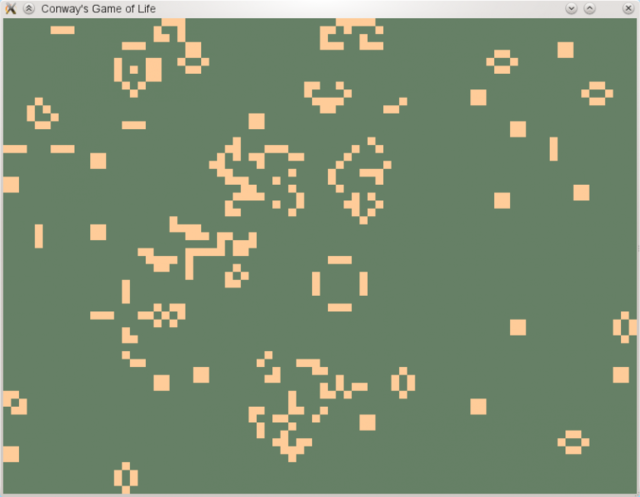# Game of Life

While reading about cellular automata in preparation for an essay it struck me that I have never actually written Conway’s Game of Life. No, really!

To correct this embarrassing fact I quickly wrote a version in Haskell using the GLUT bindings.Conway’s Game of Life

It is very simple, but it works. :-)

``````import Graphics.UI.GLUT hiding (get)
import Graphics.Rendering.GLU.Raw (gluOrtho2D)
import Data.IORef
import System.Random

-- dimensions of our cellular space
width  = 80 :: Int
height = 60 :: Int

-- takes a two-dimensional list and returns the neighbours of (x,y)
neighbours :: [[a]] -> (Int,Int) -> [a]
neighbours m (x,y) = map (\\(x',y') -> m !! y' !! x') \$ filter valid neighbours'
where height'       = length m
width'        = length (head m)
valid (x',y') = x' >= 0 && x' < width' && y' >= 0 && y' < height'
neighbours'   = [(x-1,y-1),(x,y-1),(x+1,y-1), -- neighbours over
(x-1,y),(x+1,y),             -- neighbours left/right
(x-1,y+1),(x,y+1),(x+1,y+1)] -- neighbours under

-- updates all cells according to the rules in liveOrDead
update :: IORef [[Bool]] -> IO ()
update c = do
cells <- readIORef c

let coords = [(x,y) | y <- [0..(height-1)], x <- [0..(width-1)]]

nextGen <- mapM (\\(x,y) -> do
let cell = cells !! y !! x
let ns   = neighbours cells (x,y)
return \$ liveOrDead cell ((length . filter id) ns)
) coords

writeIORef c (nLists width nextGen)
display c

-- survival rule: a live cell only lives on if it has 2 or 3 live neighbours
-- birth rule: a dead cell becomes a live cell if it has 3 live neighbours
liveOrDead :: Bool -> Int -> Bool
liveOrDead True nLive = nLive `elem` [2,3]
liveOrDead False nLive = nLive == 3

-- utility function: split a list into sublists of length n
nLists :: Int -> [a] -> [[a]]
nLists _ [] = []
nLists n ls = take n ls : nLists n (drop n ls)

-- utility function: draws a square at (x,y) with size w×h
drawQuad :: GLdouble -> GLdouble -> GLdouble -> GLdouble -> IO ()
drawQuad x y w h =
renderPrimitive Quads \$ do
vertex (Vertex3  x     y    0)
vertex (Vertex3 (x+w)  y    0)
vertex (Vertex3 (x+w) (y-h) 0)
vertex (Vertex3  x    (y-h) 0)

-- draw each cell as a coloured square
display :: IORef [[Bool]] -> IO ()
display c = do
cells <- readIORef c

let h = fromIntegral height
let w = fromIntegral width

mapM_ (\\(n,b) -> do
if b
then currentColor \$= Color4 1.0 0.8 0.6 1.0
else currentColor \$= Color4 0.4 0.5 0.4 1.0
let x = 1/w*fromIntegral (n `mod` width)
let y = 1-(1/h*fromIntegral (n `div` width))
drawQuad x y (1/w) (1/h)
) (zip [0..] \$ concat cells)

swapBuffers

main :: IO ()
main = do
g <- newStdGen
_ <- getArgsAndInitialize

-- random starting values
cells <- newIORef ((nLists width . take (width*height) . randoms) g)

_ <- createWindow "Conway's Game of Life"
initialDisplayMode    \$= [DoubleBuffered]
windowSize            \$= Size 800 600
displayCallback       \$= display cells
idleCallback          \$= Just (update cells)
gluOrtho2D 0 1 0 1    -- orthogonal projection
mainLoop              -- start main loop``````